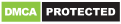# Symbolab – Math solver

Genres
Version
-
Developer
-
Requires
-
Updated
-Your private math tutor, solves any math problem with steps! Equations, integrals, derivatives, limits and much more.

Symbolab Math Solver app is composed of over one hundred of Symbolab’s most powerful calculators:
Equation Calculator
Integral Calculator
Derivative Calculator
Limit calculator
Inequality Calculator
Trigonometry Calculator
Matrix Calculator
Functions Calculator
Series Calculator
ODE Calculator
Laplace Transform Calculator